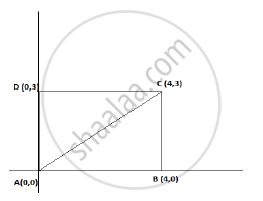Share

# ABCD is a rectangle whose three vertices are B (4, 0), C(4, 3) and D(0,3). The length of one of its diagonals is - CBSE Class 10 - Mathematics

#### Question

ABCD is a rectangle whose three vertices are B (4, 0), C(4, 3) and D(0,3). The length of one of its diagonals is
(A) 5
(B) 4
(C) 3
(D) 25

#### SolutionWe see that AB = 4 units and BC = 3 units
Using Pythagoras theorem
AC2= AB2+ BC2
=42 + 32

AC 2 =25
Thus AC = 5 units
Hence length of the diagonal of the rectangle is 5 units

Is there an error in this question or solution?

#### Video TutorialsVIEW ALL 

Solution ABCD is a rectangle whose three vertices are B (4, 0), C(4, 3) and D(0,3). The length of one of its diagonals is Concept: Pythagoras Theorem.
S